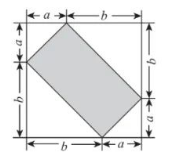$\text{A.}$ $a^2+b^2$ $\text{B.}$ $a^2-b^2$ $\text{C.}$ $2 a b$ $\text{D.}$ $4 a b$

C
①点击 首页查看更多试卷和试题 , 点击查看 本题所在试卷